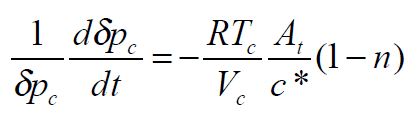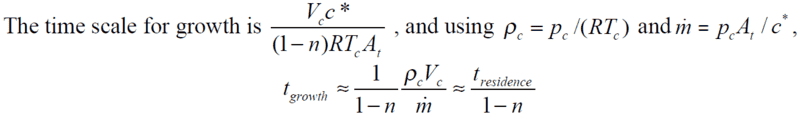Regression rate of fuel in rocket nozzleSo we have: stability for n < 1
instability for n > 1I assume to get the time growth, I have to integrate the above first eqn. However I also get ln(δpc) at the LHS from the integrating. However the above equation for time growth removed that ln(δpc) and thus I can't seem to get past this part.

Source: https://ocw.mit.edu/courses/aeronau...pring-2012/lecture-notes/MIT16_50S12_lec9.pdf

Attachments

bigfooted
Gold Member
When ##\frac{1}{y}\frac{dy}{dt}=-C## then integrating both sides leads to ## \ln(y) = -Ct ## (neglecting the constant of integration)
Taking the exponential of the left side leads to ## \exp(\ln(y)) = e^{\ln(y)} = y ##
Taking the exponential of both sides leads to ##y = \exp(-Ct) =\frac{1}{e^{Ct}}##
hope this helps.

When ##\frac{1}{y}\frac{dy}{dt}=-C## then integrating both sides leads to ## \ln(y) = -Ct ## (neglecting the constant of integration)
Taking the exponential of the left side leads to ## \exp(\ln(y)) = e^{\ln(y)} = y ##
Taking the exponential of both sides leads to ##y = \exp(-Ct) =\frac{1}{e^{Ct}}##
hope this helps.
Thanks I get this part. However the one shown on the pdf does not have the exponential for the time growth equation. How did they arrive at the above equation while getting rid of the exponential?

bigfooted
Gold Member
well, how much time does it take for ##y## to change from ##y_0## at ##t=0## to say ##2y_0##?

at ##t=0## we have ##y=\exp(0) = 1 ##, so ##y_0=1##
and at time t, we have from the equation ##\ln(y) = Ct## (I make it positive here because your equation has a negative constant):
##t = \frac{1}{C} \cdot \ln(2) ##

so to double ##y##, it takes ##t = \frac{1}{C} \cdot \ln(2) ## seconds, with a scaling factor for doubling (or tripling or whatever) of ## \frac{1}{C}##

hope this helps...